forsyde-atom-0.3.0.0: Shallow-embedded DSL for modeling cyber-physical systems

ForSyDe.Atom.Skel.Vector

Description

This module defines the data type Vector as a categorical type, and implements the atoms for the Skeleton class. Algorithmic skeletons for Vector are mostly described in their factorized form (see the factorization theorem). For practical reasons some skeletons are implemented as recurrences , but their factorized form is still documented.

Synopsis

# Vector data type

data Vector a Source #

Although the name Vector is borrowed from <ForSyDe-Atom.html#reekie95 [Reekie95]> since it is more suggestive in the context of process networks, the Vector type is in fact modeling an infinite list defined as a category in [Skillicorn05]. According to this definition it should be implemented as following:

data Vector a = Null                   -- null element
| Unit a                 -- singleton vector
| Vector a <++> Vector a -- concatenate two vectors

This construction suggests the possibility of splitting a Vector into multiple parts and evaluating it in parallel. For simplicity and to ensure that the structure is flat and homogeneous, Vector is implemented using the same constructors as a regular Haskell list (see below). When defining skeletons of vectors we will not use the real constructors though, but the theoretical ones defined above and provided as functions. This way we align ForSyDe-Atom's Vector type with the skeleton theory and its theorems.

Another particularity of Vector is that it instantiates the reduction atom =\= as a right fold, as it is the most efficient lazy implementation of lists. As a consequence reduction is performed from right to left. This is noticed especially in the case of pipeline-based skeletons (see definition of pipe as a reduction with the right-associative composition operator .) is performed from right to left. Thus for reduce-based skeletons (e.g. prefix, suffix, recur, cascade, mesh) the result vectors shall be read from end to beginning.

Constructors

 Null Null element. Terminates a vector. a :> (Vector a) infixr 3 appends an element at the head of a vector.

#### Instances

Instances details
 Source # Provides an implementation for =.=. Instance detailsDefined in ForSyDe.Atom.Skel.Vector.Core Methodsfmap :: (a -> b) -> Vector a -> Vector b #(<$) :: a -> Vector b -> Vector a # Source # Provides an implementation for =*=. Instance detailsDefined in ForSyDe.Atom.Skel.Vector.Core Methodspure :: a -> Vector a #(<*>) :: Vector (a -> b) -> Vector a -> Vector b #liftA2 :: (a -> b -> c) -> Vector a -> Vector b -> Vector c #(*>) :: Vector a -> Vector b -> Vector b #(<*) :: Vector a -> Vector b -> Vector a # Source # Provides an implementation for =\=. Instance detailsDefined in ForSyDe.Atom.Skel.Vector.Core Methodsfold :: Monoid m => Vector m -> m #foldMap :: Monoid m => (a -> m) -> Vector a -> m #foldMap' :: Monoid m => (a -> m) -> Vector a -> m #foldr :: (a -> b -> b) -> b -> Vector a -> b #foldr' :: (a -> b -> b) -> b -> Vector a -> b #foldl :: (b -> a -> b) -> b -> Vector a -> b #foldl' :: (b -> a -> b) -> b -> Vector a -> b #foldr1 :: (a -> a -> a) -> Vector a -> a #foldl1 :: (a -> a -> a) -> Vector a -> a #toList :: Vector a -> [a] #null :: Vector a -> Bool #length :: Vector a -> Int #elem :: Eq a => a -> Vector a -> Bool #maximum :: Ord a => Vector a -> a #minimum :: Ord a => Vector a -> a #sum :: Num a => Vector a -> a #product :: Num a => Vector a -> a # Source # Ensures that Vector is a structure associated with the Skeleton Layer. Instance detailsDefined in ForSyDe.Atom.Skel.Vector.Core Methods(=.=) :: (a -> b) -> Vector a -> Vector b Source #(=*=) :: Vector (a -> b) -> Vector a -> Vector b Source #(=\=) :: (a -> a -> a) -> Vector a -> a Source #(=<<=) :: Vector (a -> a) -> a -> a Source #first :: Vector a -> a Source #last :: Vector a -> a Source # Eq a => Eq (Vector a) Source # Instance detailsDefined in ForSyDe.Atom.Skel.Vector.Core Methods(==) :: Vector a -> Vector a -> Bool #(/=) :: Vector a -> Vector a -> Bool # Read a => Read (Vector a) Source # The vector 1 :> 2 :> Null is read using the string "<1,2>". Instance detailsDefined in ForSyDe.Atom.Skel.Vector.Core MethodsreadsPrec :: Int -> ReadS (Vector a) #readList :: ReadS [Vector a] # Show a => Show (Vector a) Source # The vector 1 :> 2 :> Null is represented as <1,2>. Instance detailsDefined in ForSyDe.Atom.Skel.Vector.Core MethodsshowsPrec :: Int -> Vector a -> ShowS #show :: Vector a -> String #showList :: [Vector a] -> ShowS # Plottable a => Plottable (Vector a) Source # Vectors of plottable types Instance detailsDefined in ForSyDe.Atom.Utility.Plot Methods Plottable a => Plot (Vector a) Source # For plotting vectors of coordinates Instance detailsDefined in ForSyDe.Atom.Utility.Plot Methodssample :: Float -> Vector a -> Samples Source #takeUntil :: Float -> Vector a -> Vector a Source # # "Constructors" Theoretical constructors for the Vector type, used in the definition of skeletons as catamorphisms. Constructs a null vector. >>> null <>  unit :: a -> Vector a Source # Constructs a singleton vector. >>> unit 1 <1>  (<++>) :: Vector a -> Vector a -> Vector a infixr 5 Source # Constructs a vector by appending two existing vectors. >>> unit 1 <++> unit 2 <1,2>  # Utilities vector :: [a] -> Vector a Source # Converts a list to a vector. fromVector :: Vector a -> [a] Source # Converts a vector to a list. Creates the infinite vector: <1,2,3,4,...> Used mainly for operation on indexes. isNull :: Vector a -> Bool Source # Returns True if the argument is a null vector. (<:) :: Vector a -> a -> Vector a infixl 5 Source # Appends an element at the end of a vector. # Skeletons Algorithmic skeletons on vectors are mainly presented in terms of compositions of the atoms associated with the Skeleton Layer. When defining them, we use the following operators:where: • (1) is the unit constructor, constructing a singleton vector. • (2) is the <++> constructor, concatenating two vectors. • (3) is the <@! selector. The subscript notation is used to denote element at position n in a vector. • (4) suggests an arbitrary selector which returns a vector with another one's elements, based on some indices. The shown example is an alternative notation for the tail skeleton. ## Functional networks This sub-category denotes skeletons (patterns) which are take functions as arguments. If the functions are MoC layer entities, i.e. processes, then these patterns are capable of constructing parallel process networks. Using the applicative mechanism, the designer has a high degree of freedom when customizing process networks through systematic partial application, rendering numerous possible usages for the same pattern. To avoid over-encumbering the figures, they depict small test cases, which might not expose the full potential of the constructors. see the naming convention rules on how to interpret, use and develop your own constructors. Arguments  :: (a1 -> a2 -> (b1, b2)) function (e.g. process) -> Vector a1 first input vector -> Vector a2 second input vector -> (Vector b1, Vector b2) two output vectors farm is simply the Vector instance of the skeletom farm pattern (see farm22). If the function taken as argument is a process, then it creates a farm network of data parallel processes. Constructors: farm[1-4][1-4]. >>> let v1 = vector [1,2,3,4,5] >>> S.farm21 (+) v1 v1 <2,4,6,8,10> >>> let s1 = SY.signal [1,2,3,4,5] >>> let v2 = vector [s1,s1,s1] >>> S.farm11 (comb11 (+1)) v2 <{2,3,4,5,6},{2,3,4,5,6},{2,3,4,5,6}> >>> S.farm21 (\x -> comb11 (+x)) v1 v2 <{2,3,4,5,6},{3,4,5,6,7},{4,5,6,7,8}>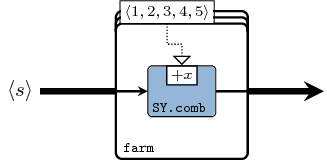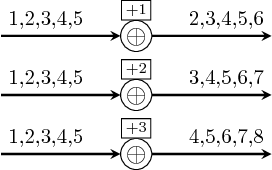reduce :: (a -> a -> a) -> Vector a -> a Source # As the name suggests, it reduces a vector to an element based on an associative function. If the function is not associative, it can be treated like a pipeline. Vector instantiates the skeletons for both reduce and reducei. >>> let v1 = vector [1,2,3,4,5] >>> S.reduce (+) v1 15 >>> let s1 = SY.signal [1,2,3,4,5] >>> let s2 = SY.signal [10,10,10,10,10] >>> let v2 = vector [s1,s1,s1] >>> S.reduce (comb21 (+)) v2 {3,6,9,12,15} >>> S.reducei (comb21 (+)) s2 v2 {13,16,19,22,25}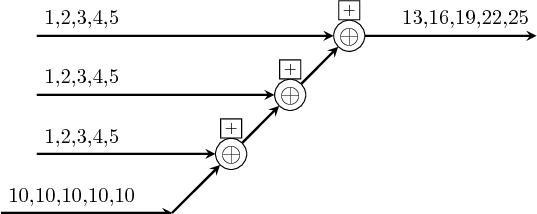prefix :: (b -> b -> b) -> Vector b -> Vector b Source # prefix peforms the parallel prefix operation on a vector. Equivalent process networks are constructed if processes are passed as arguments. Similar to reduce and reducei, two versions prefix and prefixi are provided. >>> let v1 = vector [1,2,3,4,5] >>> prefix (+) v1 <15,14,12,9,5> >>> let s1 = SY.signal [1,2,3,4,5] >>> let s2 = SY.signal [10,10,10,10,10] >>> let v2 = vector [s1,s1,s1] >>> prefix (comb21 (+)) v2 <{3,6,9,12,15},{2,4,6,8,10},{1,2,3,4,5}> >>> prefixi (comb21 (+)) s2 v2 <{13,16,19,22,25},{12,14,16,18,20},{11,12,13,14,15}>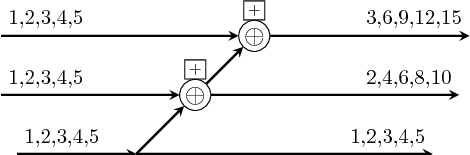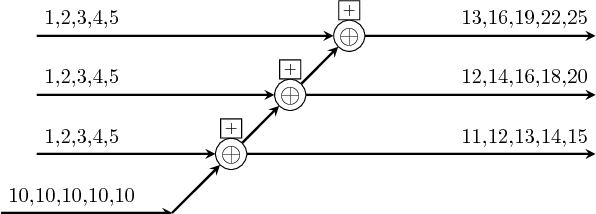suffix :: (b -> b -> b) -> Vector b -> Vector b Source # suffix peforms the parallel suffix operation on a vector. Equivalent process networks are constructed if processes are passed as arguments. Similar to reduce and reducei, two versions suffix and suffixi are provided. >>> let v1 = vector [1,2,3,4,5] >>> suffix (+) v1 <1,3,6,10,15> >>> let s1 = SY.signal [1,2,3,4,5] >>> let s2 = SY.signal [10,10,10,10,10] >>> let v2 = vector [s1,s1,s1] >>> suffix (comb21 (+)) v2 <{1,2,3,4,5},{2,4,6,8,10},{3,6,9,12,15}> >>> suffixi (comb21 (+)) s2 v2 <{11,12,13,14,15},{12,14,16,18,20},{13,16,19,22,25}>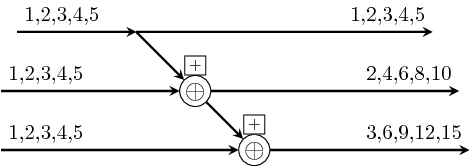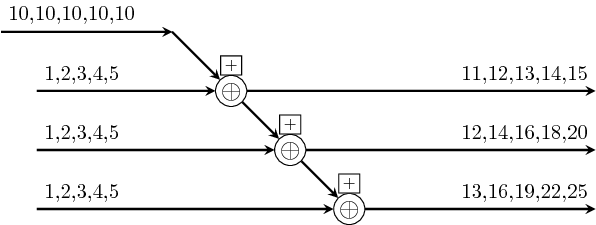Arguments  :: Vector (a -> a) vector of functions -> a input -> a output pipe creates a pipeline of functions from a vector. pipe simply instantiates the =<<= atom whereas pipeX instantiate their omologi from the ForSyDe.Atom.Skel module (see pipe2). OBS: the pipelining is done in the order dictated by the function composition operator: from right to left. Constructors: pipe[1-4]. >>> let v1 = vector [(+1),(+1),(+1)] >>> S.pipe v1 1 4 >>> let s1 = SY.signal [1,2,3,4] >>> let v2 = vector [1,2,3,4] >>> S.pipe1 (\x -> comb11 (+x)) v2 s1 {11,12,13,14}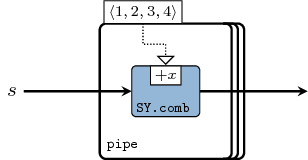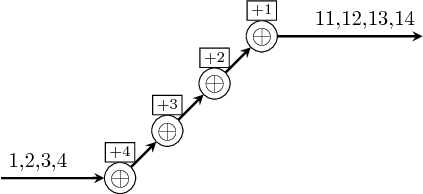(=/=) :: Vector (a -> a) -> a -> Vector a infixl 2 Source # Infix operator for recur. Arguments  :: Vector (a -> a) vector of functions -> a input -> Vector a output recur creates a systolic array from a vector of functions. Just like pipe and pipeX, there exists a raw recur version with an infix operator =/=, and the enhanced recurX which is meant for systematic partial application of a function on an arbitrary number of vectors until the desired vector of functions is obtained. Constructors: (=/=), recur, recuri, recur[1-4][1-4]. >>> let v1 = vector [(+1),(+1),(+1)] >>> recur v1 1 <4,3,2> >>> recuri v1 1 <4,3,2,1> >>> let s1 = SY.signal [1,2,3,4] >>> let v2 = vector [1,2,3,4] >>> recur1 (\x -> comb11 (+x)) v2 s1 <{11,12,13,14},{10,11,12,13},{8,9,10,11},{5,6,7,8}>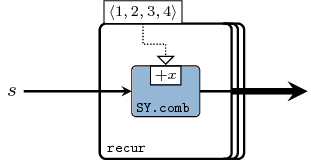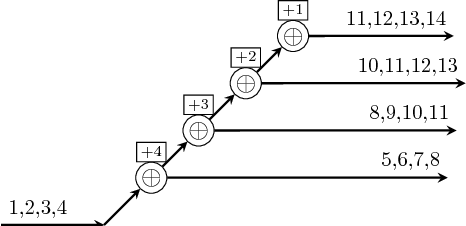Arguments  :: (a2 -> a1 -> a -> a -> a) function41 which needs to be applied to function21 -> Vector (Vector a2) fills in the first argument in the function above -> Vector (Vector a1) fills in the second argument in the function above -> Vector a first input vector (e.g. of signals) -> Vector a second input vector (e.g. of signals) -> Vector a output cascade creates a "cascading mesh" as a result of piping a vector into a vector of recur arrays. Constructors: cascade, cascade[1-4]. >>> let v1 = vector [1,2,3,4] >>> cascade (+) v1 v1 <238,119,49,14> >>> let s1 = SY.signal [1,2,3,4] >>> let vs = vector [s1, s1, s1] >>> cascade (comb21 (+)) vs vs <{20,40,60,80},{10,20,30,40},{4,8,12,16}> >>> let vv = vector [vector [1,-1,1], vector [-1,1,-1], vector [1,-1,1] ] >>> cascade1 (\x -> comb21 (\y z-> x*(y+z))) vv vs vs <{16,32,48,64},{8,16,24,32},{-2,-4,-6,-8}>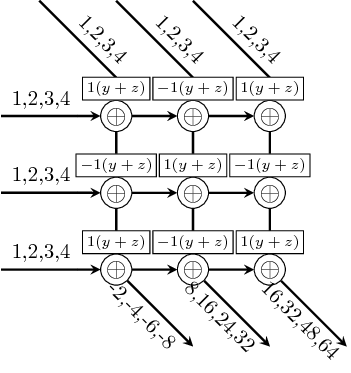Arguments  :: (a2 -> a1 -> a -> a -> a) function41 which needs to be applied to function21 -> Vector (Vector a2) fills in the first argument in the function above -> Vector (Vector a1) fills in the second argument in the function above -> Vector a first input vector (e.g. of signals) -> Vector a second input vector (e.g. of signals) -> Vector (Vector a) output, a 2D vector mesh creates a 2D systolic array as a result of piping a vector into a vector of 1D systolic arrays. Constructors: mesh, mesh[1-4]. >>> let v1 = vector [1,2,3,4] >>> mesh (+) v1 v1 <<238,119,49,14>,<119,70,35,13>,<49,35,22,11>,<14,13,11,8>> >>> let s1 = SY.signal [1,2,3,4] >>> let vs = vector [s1, s1, s1] >>> mesh (comb21 (+)) vs vs <<{20,40,60,80},{10,20,30,40},{4,8,12,16}>,<{10,20,30,40},{6,12,18,24},{3,6,9,12}>,<{4,8,12,16},{3,6,9,12},{2,4,6,8}>> >>> let vv = vector [vector [1,-1,1], vector [-1,1,-1], vector [1,-1,1]] >>> mesh1 (\x -> comb21 (\y z-> x*(y+z))) vv vs vs <<{16,32,48,64},{8,16,24,32},{-2,-4,-6,-8}>,<{8,16,24,32},{-6,-12,-18,-24},{-3,-6,-9,-12}>,<{-2,-4,-6,-8},{-3,-6,-9,-12},{2,4,6,8}>>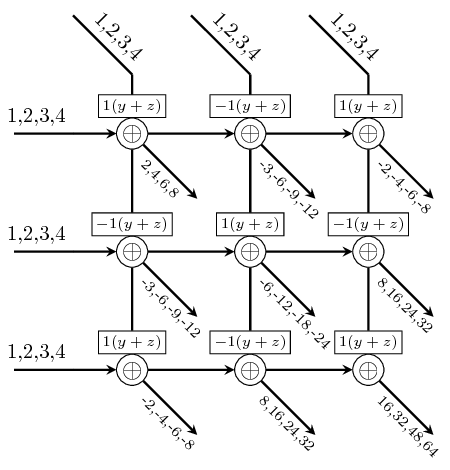## Queries Queries return various information about a vector. They are also built as skeletons. length :: Num p => Vector a -> p Source # returns the number of elements in a value. >>> length$ vector [1,2,3,4,5]
5index :: Vector a2 -> Vector Int Source #

returns a vector with the indexes from another vector.

>>> index $vector [1,1,1,1,1,1,1] <1,2,3,4,5,6,7>  ## Generators Generators are specific applications of the prefix or suffix skeletons. fanout :: t -> Vector t Source # fanout repeats an element. As a process network it distributes the same value or signal to all the connected processes down the line. Depending on the target platform and the refinement decisions involved, it may be interpreted in the following implementations: • global or shared memory in case of a massively parallel platform (e.g. GPU) • a (static) memory or cache location in memory-driven architectures (e.g. CPU) • a fanout in case of a HDL system • a broadcast in case of a distributed system fanoutn :: (Ord t, Num t) => t -> a -> Vector a Source # fanoutn is the same as fanout, but the length of the result is also provided. >>> fanoutn 5 1 <1,1,1,1,1>  generate :: (Ord t, Num t) => t -> (a -> a) -> a -> Vector a Source # generate creates a vector based on a kernel function. It is just a restricted version of recur. >>> generate 5 (+1) 1 <6,5,4,3,2>iterate :: (Ord t, Num t) => t -> (a -> a) -> a -> Vector a Source # iterate is a version of generate which keeps the initial element as well. It is a restricted version of recuri. >>> iterate 5 (+1) 1 <5,4,3,2,1>  ## Permutators Permutators perform operations on the very structure of vectors, and make heavy use of the vector constructors. first :: Vector a -> a Source # Instance of first >>> S.first$ vector [1,2,3,4,5]
1


last :: Vector a -> a Source #

Instance of last

>>> S.last $vector [1,2,3,4,5] 5  inits :: Vector a -> Vector (Vector a) Source # creates a vector of all the initial segments in a vector. >>> inits$ vector [1,2,3,4,5]
<<1>,<1,2>,<1,2,3>,<1,2,3,4>,<1,2,3,4,5>>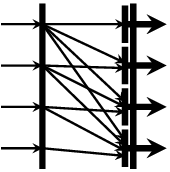tails :: Vector a -> Vector (Vector a) Source #

creates a vector of all the final segments in a vector.

>>> tails $vector [1,2,3,4,5] <<1,2,3,4,5>,<2,3,4,5>,<3,4,5>,<4,5>,<5>>init :: Vector a -> Vector a Source # Returns the initial segment of a vector. >>> init$ vector [1,2,3,4,5]
<1,2,3,4>tail :: Vector a -> Vector a Source #

Returns the tail of a vector.

>>> tail $vector [1,2,3,4,5] <2,3,4,5>concat :: Vector (Vector a) -> Vector a Source # concatenates a vector of vectors. >>> concat$ vector [vector[1,2,3,4], vector[5,6,7]]
<1,2,3,4,5,6,7>reverse :: Vector a -> Vector a Source #

reverses the elements in a vector.

>>> reverse $vector [1,2,3,4,5] <5,4,3,2,1>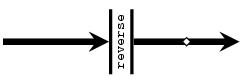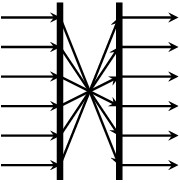group :: Int -> Vector a -> Vector (Vector a) Source # groups a vector into sub-vectors of n elements. >>> group 3$ vector [1,2,3,4,5,6,7,8]
<<1,2,3>,<4,5,6>,<7,8>>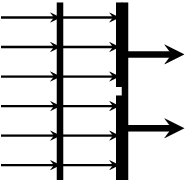shiftr :: Vector a -> a -> Vector a Source #

right-shifts a vector with an element.

>>> vector [1,2,3,4] shiftr 8
<8,1,2,3>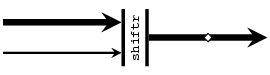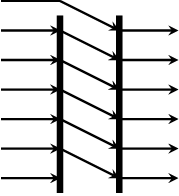shiftl :: Vector a -> a -> Vector a Source #

left-shifts a vector with an element.

>>> vector [1,2,3,4] shiftl 8
<2,3,4,8>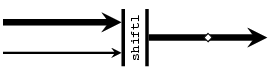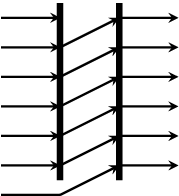rotr :: Vector a -> Vector a Source #

rotates a vector to the right.

>>> rotr $vector [1,2,3,4] <4,1,2,3>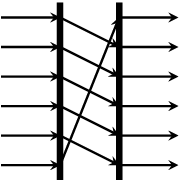rotl :: Vector a -> Vector a Source # rotates a vector to the left. >>> rotl$ vector [1,2,3,4]
<2,3,4,1>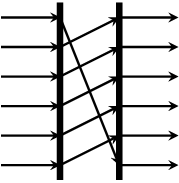rotate :: Int -> Vector a -> Vector a Source #

rotates a vector to the left or to the right depending on the index:

• (> 0) : rotates the vector right with the corresponding number of positions.
• (= 0) : does not modify the vector.
• (< 0) : rotates the vector left with the corresponding number of positions.

take :: Int -> Vector a -> Vector a Source #

takes the first n elements of a vector.

>>> take 5 $vector [1,2,3,4,5,6,7,8,9] <1,2,3,4,5>drop :: Int -> Vector a -> Vector a Source # drops the first n elements of a vector. >>> drop 5$ vector [1,2,3,4,5,6,7,8,9]
<6,7,8,9>takeWhile :: (a -> Bool) -> Vector a -> Vector a Source #

takes the first elements in a vector until the first element that does not fulfill a predicate.

>>> takeWhile (<5) $vector [1,2,3,4,5,6,7,8,9] <1,2,3,4>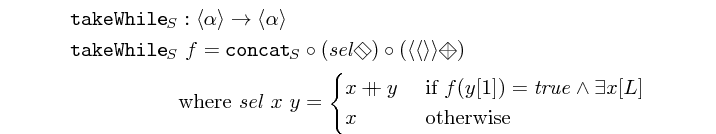filterIdx :: (Int -> Bool) -> Vector a -> Vector a Source # returns a vector containing only the elements of another vector whose index satisfies a predicate. >>> filterIdx (\x -> x mod 3 == 0)$ vector [0,1,2,3,4,5,6,7,8,9]
<2,5,8>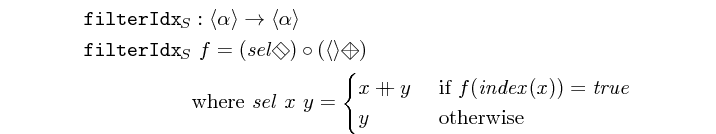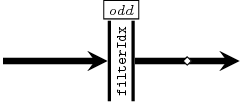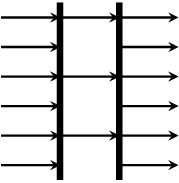odds :: Vector a -> Vector a Source #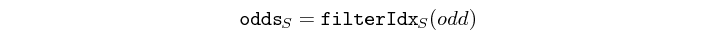evens :: Vector a -> Vector a Source #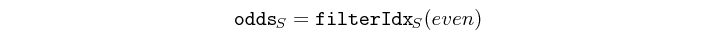Arguments

 :: Int first index -> Int stride length -> Vector a -> Vector a

does a stride-selection on a vector.

>>> stride 1 3 $vector [1,2,3,4,5,6,7,8,9] <1,4,7>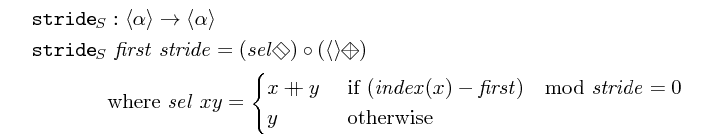get :: Int -> Vector a -> Maybe a Source # returns the n-th element in a vector, or Nothing if n > l. >>> get 3$ vector [1,2,3,4,5]
Just 3(<@) :: Vector a -> Int -> Maybe a Source #

the same as get but with flipped arguments.

(<@!) :: Vector p -> Int -> p Source #

unsafe version of <@. Throws an exception if n > l.

Arguments

 :: Vector Int vector of indexes -> Vector a input vector -> Vector (Maybe a)

selects the elements in a vector at the incexes contained by another vector.

The following versions of this skeleton are available, the number suggesting how many nested vectors it is operating upon: gather[1-5]

>>> let ix = vector [vector [1,3,4], vector [3,5,1], vector [5,8,9]]
>>> let v = vector [11,12,13,14,15]
>>> gather2 ix v
<<Just 11,Just 13,Just 14>,<Just 13,Just 15,Just 11>,<Just 15,Nothing,Nothing>>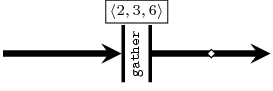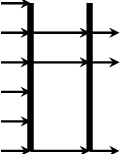Arguments

 :: Vector a input vector -> Vector Int vector of indexes -> Vector (Maybe a)

the same as gather1 but with flipped arguments

The following versions of this skeleton are available, the number suggesting how many nested vectors it is operating upon.

(<@>), (<<@>>), (<<<@>>>), (<<<<@>>>>), (<<<<<@>>>>>),

replace :: Int -> a -> Vector a -> Vector a Source #

replaces the n-th element in a vector with another.

>>> replace 5 15 \$ vector [1,2,3,4,5,6,7,8,9]
<1,2,3,4,15,6,7,8,9>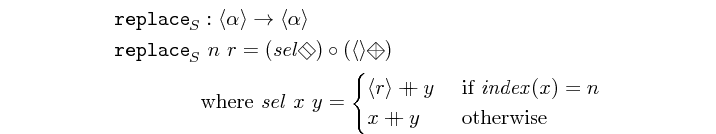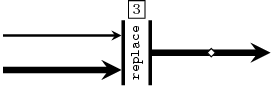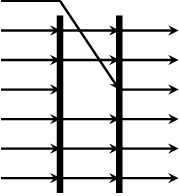scatter :: Vector Int -> Vector a -> Vector a -> Vector a Source #

scatters the elements in a vector based on the indexes contained by another vector.

>>> scatter (vector [2,4,5]) (vector [0,0,0,0,0,0,0,0]) (vector [1,1,1])
<0,1,0,1,1,0,0,0>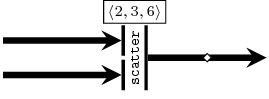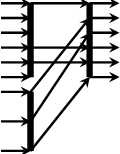## Interfaces

Arguments

 :: MoC e => Vector ((Vector a -> Vector a -> Vector a) -> Fun e (Vector a) (Fun e (Vector a) (Ret e (Vector a)))) vector of MoC-specific context wrappers for the function <++> -> Vector (Stream (e a)) input vector of signals -> Stream (e (Vector a)) output signal of vectors

zipx is a template skeleton for "zipping" a vector of signals. It synchronizes all signals (of the same MoC) in a vector and outputs one signal with vectors of the synced values. For each signal in the input vector it requires a function which translates a partition of events (see ForSyDe.Atom.MoC) into sub-vectors.

There exist helper instances of the zipx skeleton interface for all supported MoCs.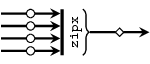unzipx :: MoC e => (Vector a -> Vector (Ret e a)) -> Integer -> Stream (e (Vector a)) -> Vector (Stream (e a)) Source #

unzipx is a template skeleton to unzip a signal carrying vectors into a vector of multiple signals. It required a function that splits a vector of values into a vector of event partitions belonging to output signals. Unlike zipx, it also requires the number of output signals. The reason for this is that it is impossible to determine the length of the output vector without "sniffing" the content of the input events, which is out of the scope of skeletons and may lead to unsafe behavior. The length of the output vector is needed in order to avoid infinite recurrence.

There exist helper instances of the unzipx skeleton interface for all supported MoCs.